Taylor polynomials: Rational function 1

The rational functionis, as in the example of the square root, a special case of Newton's Binomial Theorem. We can calculate its Taylor's series at x = 0 easily.

This function has a singularity at the point x =- 1. The approximation is good in ranges from -1 to +1. Again we find a useful approach that is centered on the origin.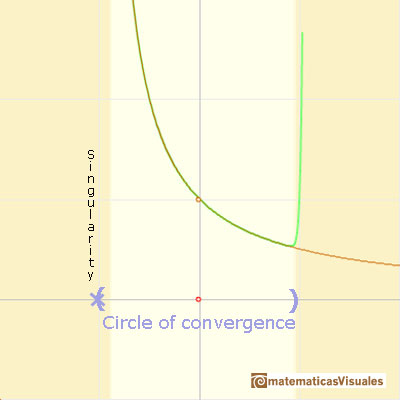At the point x = +1 their ordinates are alternately equal to 1 and 0, while that of the original curve has the value 1/2.We can compare this behavior with that of the rational function 2.

REFERENCES

Felix Klein - Elementary Mathematics from an Advanced Standpoint. Arithmetic, Algebra, Analysis (pags. 223-228) - Dover Publications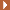NEXT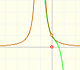The function has a singularity at -1. Taylor polynomials about the origin approximates the function between -1 and 1.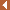PREVIOUSThe function is not defined for values less than -1. Taylor polynomials about the origin approximates the function between -1 and 1.This function has two real singularities at -1 and 1. Taylor polynomials approximate the function in an interval centered at the center of the series. Its radius is the distance to the nearest singularity.This is a continuos function and has no real singularities. However, the Taylor series approximates the function only in an interval. To understand this behavior we should consider a complex function.Mercator published his famous series for the Logarithm Function in 1668. Euler discovered a practical series to calculate.We will see how Taylor polynomials approximate the function inside its circle of convergence.By increasing the degree, Taylor polynomial approximates the exponential function more and more.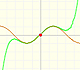By increasing the degree, Taylor polynomial approximates the sine function more and more.The derivative of a lineal function is a constant function.The derivative of a quadratic function is a linear function, it is to say, a straight line.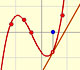The derivative of a cubic function is a quadratic function, a parabola.Lagrange polynomials are polynomials that pases through n given points. We use Lagrange polynomials to explore a general polynomial function and its derivative.